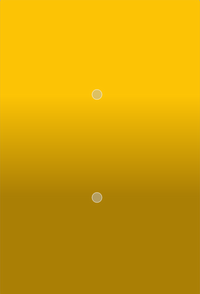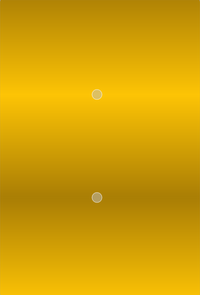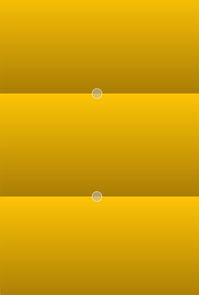The `QLinearGradient` class is used in combination with `QBrush` to specify a linear gradient brush. More

## Synopsis#

### Functions#

Note

This documentation may contain snippets that were automatically translated from C++ to Python. We always welcome contributions to the snippet translation. If you see an issue with the translation, you can also let us know by creating a ticket on https:/bugreports.qt.io/projects/PYSIDE

## Detailed Description#

Linear gradients interpolate colors between start and end points. Outside these points the gradient is either padded, reflected or repeated depending on the currently set `spread` method:`PadSpread` (default) `ReflectSpread` `RepeatSpread`

The colors in a gradient is defined using stop points of the `QGradientStop` type, i.e. a position and a color. Use the `setColorAt()` or the `setStops()` function to define the stop points. It is the gradient’s complete set of stop points that describes how the gradient area should be filled. If no stop points have been specified, a gradient of black at 0 to white at 1 is used.

In addition to the functions inherited from `QGradient` , the `QLinearGradient` class provides the `finalStop()` function which returns the final stop point of the gradient, and the `start()` function returning the start point of the gradient.

Parameters:

Constructs a default linear gradient with interpolation area between (0, 0) and (1, 1).

Constructs a linear gradient with interpolation area between the given `start` point and `finalStop`.

Note

The expected parameter values are in pixels.

Constructs a linear gradient with interpolation area between (`x1`, `y1`) and (`x2`, `y2`).

Note

The expected parameter values are in pixels.

Return type:

`PySide6.QtCore.QPointF`

Returns the final stop point of this linear gradient in logical coordinates.

Parameters:

Sets the final stop point of this linear gradient in logical coordinates to `stop`.

Parameters:
• x – float

• y – float

Sets the final stop point of this linear gradient in logical coordinates to `x`, `y`.

Parameters:

Sets the start point of this linear gradient in logical coordinates to `start`.

Parameters:
• x – float

• y – float

Sets the start point of this linear gradient in logical coordinates to `x`, `y`.
`PySide6.QtCore.QPointF`